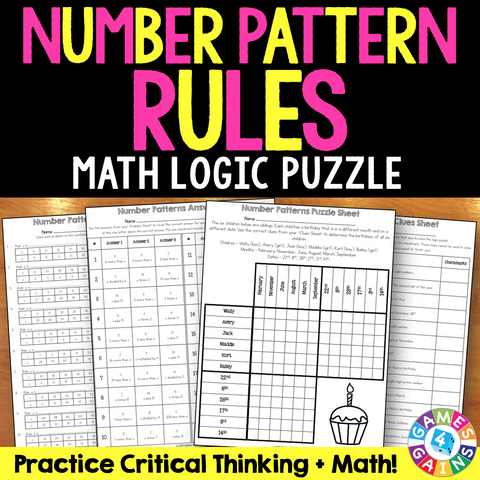## Number Pattern Rules Logic Puzzle (5.OA.3)

• \$200

Engage your students in practicing both their math skills and their higher level thinking skills with this Number Pattern Rules Logic Puzzle! This activity is sure to make determining the rule between two sets of numbers fun!

For this activity, students answer all of the math problems to determine which clues are "valid" to use for solving the logic puzzle. Then, they must use those clues to solve the logic puzzle correctly. The logic puzzle is a great way to motivate students to work through each problem.

This activity is meant to support Common Core 5.OA.B.3: Generate two numerical patterns using two given rules. Identify apparent relationships between corresponding terms.

Included with this Number Pattern Rules Logic Puzzle:
• Directions for use
• Problems sheet (20 problems)